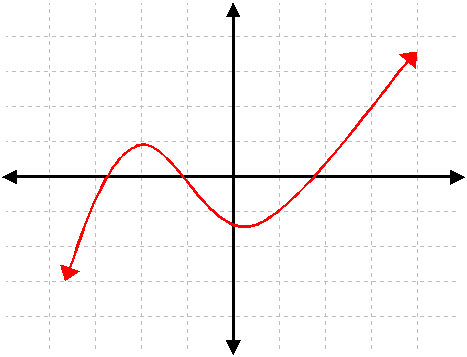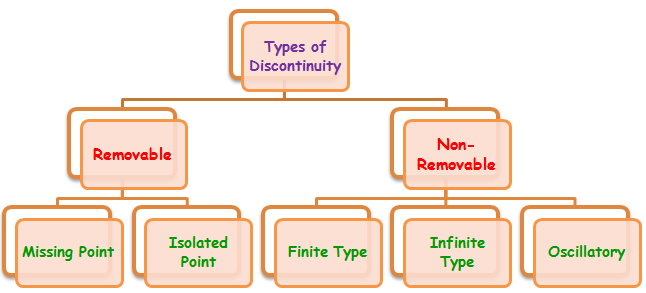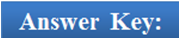Click to Chat

1800-1023-196

+91-120-4616500

CART 0

• 0

MY CART (5)

Use Coupon: CART20 and get 20% off on all online Study Material

ITEM
DETAILS
MRP
DISCOUNT
FINAL PRICE
Total Price: Rs.

There are no items in this cart.
Continue Shopping```Discontinuity

Table of Content

Important Points

Removable Discontinuity

Missing Point Discontinuity

Non-Removable Discontinuity

Related ResourcesWe have already discussed the concept of limits and continuity in the previous sections. Limits, continuity and differentiability are the building blocks of Differential Calculus. They lay the foundation for all other topics.

A function f(x) is said to be continuous at x = a if

lim x→a- f(x) = lim x→a+ f(x) = f(a).

Thus, unlike limits, for continuity it is essential for the function to be defined at that particular point and the limiting value of the function should be equal to f(a).

The function f(x) will be discontinuous at x = a in either of the following situations:1. lim x→a- f(x) and lim x→a+ f(x) exist but are not equal.

2. lim x→a- f(x) and lim x→a+ f(x) exist and are equal but not equal to f(a).

3. f(a) is not defined.

4. At least one of the limits does not exist.

Important Points

Every polynomial function is continuous at every point of the real line.

f(x) = a0xn + a1xn-1 + …. an, ∀ x ∈ R.

Every rational function si continuous at every point where its denominator is different from zero,

Logarithmic functions, exponential functions, trigonometric functions, inverse circular functions and modulus functions are continuous in their respective domains.

A function having finite number of jumps in a given domain is piecewise continuous.

There can be various types of discontinuities. The chart given below illustrates the basic types of discontinuities:We now discuss each of these discontinuities in detail one by one along with various examples:

Removable Discontinuity

In case of removable discontinuity, the limit of the function i.e. lim x→a f(x) necessarily exists but the problem is that it is either not equal to the value of the function at ‘a’ i.e. f(a) or f(a) is not defined. However, it is therefore possible to redefine the function in such a way that the limit equals the value of the function at that particular point i.e. lim x→a f(x) = f(a).

Now, removable discontinuity can further be divided into missing point discontinuity and isolated point discontinuity.

Illustration:

Redefine the function f(x) = [x] + [-x] in such a way that it becomes continuous for x ∈ (0, 2).

Solution:

Here lim x→1 f(x) = -1 but f(a) = 0

Hence, f(x) has a removable discontinuity at x = 1.

To remove this we define f(x) as follows

f(x) = [x] + [-x], x ∈ (0, 1) U (1, 2)

= -1, x = 1.

Now, f(x) is continuous for x ∈ (0, 2).

Missing Point Discontinuity

The discontinuity is said to be of missing point type if the limit of the function exists at point ‘a’ but the function is not defined at that point, i.e. lim x→a f(x) exists but f(a) is not defined.Consider the function f(x) = (x2 - 4)/(x – 2), where x ≠ 2.

It is visible from the graph as well as the definition of the function that f(2) is not defined and hence, f(x) has missing point of discontinuity at x = 2.

Isolated Point Discontinuity

In this type of discontinuity, not only does the limit of the function exist at the particular point but the function is also defined at that point, but both are not equal, i.e. lim x→a f(x) exists but lim x→a f(x) ≠ f(a).

Example: Consider the function

f(x) = sgn(cos 2x – 2sin x + 3) = sgn (2 (2 + sin x)(1 – sin x)) = 0, if x = 2nπ + π/2

= +1, if x ≠ 2nπ + π/2

has an isolated point at x = 0 discontinuity as x = 2nπ + π/2.

View the following video on types of discontinuities:

Non-Removable Discontinuity

A function is said to possess non-removable discontinuity if the limit of the function does not exist. Thus, since lim x→a f(x) does not exist therefore it is not possible to redefine the function in any way so as to make it continuous. The non-removable discontinuities can further be classified into three heads:

(a) Finite Type:

In this type of discontinuity, both the left and right hand limits exist but are unequal. We consider certain examples in this context:

(1) lim x→0 tan-1(1/x), now consider the limits

f(0+) = π/2

f(0-) = – π/2

Hence, the jump in this case is π.

(2) lim x→0 |sin x|/x

f(0+) = 1

f(0-) = – 1

Hence, the jump in this case is 2.

The non-negative difference between the two limits is called the Jump of Discontinuity.

Illustration :

Consider f(x) =[x], where [x] is greatest integer < x.

Solution:

Consider x =1. Where I is some integer

RHL = limx→1+ f(x) = 1 and

LHL = limx→a-  f(x) = 1-1

Hence both RHL and LHL are finite real numbers but they are not equal.

______________________________________________________________

A function possessing a finite number of jumps in a given finite interval is called a piecewise continuous function.(b) Infinite Type:

The discontinuity is said to be of infinite type if at least one of the two limits is infinity. We illustrate this concept with the help of following examples:

(1) f(x) = x/(x – 1) at x = 1

f(1+) = – ∞

f(1-) = + ∞

(2) f(x) = x/(x – 1) at x = 0

f(0+) = ∞

f(0-) = ∞

(c) Oscillatory:

The discontinuity is said to be oscillatory when the limits oscillate between two finite quantities.

In case of trigonometric functions, say f(x) = cos 1/x or sin 1/x, at x = 0, the limit oscillates between -1 and 1.

We now discuss certain illustrations based on the discussed concepts:

Illustration 1:

The function

f(x) = [log(1 + ax) – log(1 – bx)]/ x

is not defined at x = 0. The value which should be assigned to f at x = 0 so that it is continuous at 0 is... ? (IIT JEE 1983)

(a) a-b                                                               (b) a+b

(c) log a + log b                                                  (d) None of these

Solution:

We know for the function f to be continuous we must have

f(0) = lim x→0 f(x)

= lim x→0 [log (1 + ax) – log(1 – bx)]/ x

= lim x→0 a[log(1 + ax)/ax + b log(1 – bx)]/ -bx

= a.1 + b.1

= a + b (since f(0) = a + b).

_____________________________________________________________________

Illustration 2:

The function f(x) = [x]2 – [x2] is discontinuous at (IIT JEE 1999)

(a) all integers

(b) all integers except 0 and 1

(c) all integers except 0

(d) all integers except 1

Solution:

This question is based on greatest integer function. We know that all integers are critical points for the greatest integer function.

Case 1: When x ∈ I

f(x) = [x]2 – [x2] = x2 –x2 = 0

Case 2: When x does not belong to I

If 0 < x < 1, then [x] = 0

And 0 < x2 < 1, then [x2] = 0

Next, if 1 ≤ x2 < 2

Then 1 ≤ x < √2

So, [x] = 1 and [x2] = 1

Therefore, f(x) = [x]2 – [x2] = 0 if 1 ≤ x <√2

Hence, f(x) = 0 if 0 ≤ x < √2

This shows that f(x) is continuous at x = 1.

Hence f(x) is discontinuous in (-∞, 0) ∪[√2, ∞) on many other points.Q1. In case of a discontinuous function, which of the following is false?

(a) lim x→a- f(x) and lim x→a+ f(x) exist but are not equal.

(b) lim x→a- f(x) and lim x→a+ f(x) exist and are equal but not equal to f(a).

(c) f(a) is not defined.

(d) none of these

Q2. Which of the following functions are continuous in their domains?

(a) polynomial, exponential and logarithmic functions

(b) signum function

(c) signum and polynomial functions

(d) none of these

Q3. The product of a continuous and a discontinuous function is

(a) necessarily continuous

(b) may or may not be discontinuous

(c) necessarily discontinuous

(d) none of these

Q4. Removable discontinuity can further be classified as

(a) missing point and oscillatory discontinuity

(b) isolated point and mixed discontinuity

(c) missing point and isolated point discontinuity

(d) mixed discontinuity and missing point discontinuity

Q5. Which of the following statements is true?

(a) discontinuity implies non-derivability

(b) continuity implies derivability

(c) non-derivability implies discontinuity

(d) none of theseQ1.

Q2.

Q3.

Q4.

Q5.

(d)

(a)

(b)

(c)

(a)

Related Resources

You may wish to refer indefinite integral.

For getting an idea of the type of questions asked, refer the previous year papers.

To read more, Buy study materials of Limtis and Continuity comprising study notes, revision notes, video lectures, previous year solved questions etc. Also browse for more study materials on Mathematics here.

```### Course Features

• 731 Video Lectures
• Revision Notes
• Previous Year Papers
• Mind Map
• Study Planner
• NCERT Solutions
• Discussion Forum
• Test paper with Video Solution